# 28. CURRENTS AND OHMS LAW

## 28.1. Electric Current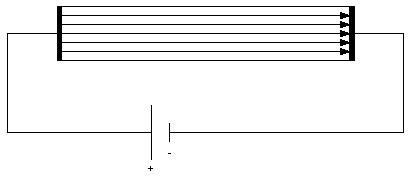Figure 28.1. Electric field in a wire.

When a wire is connected to the terminals of a battery, an electric field is generated inside the wire (see Figure 28.1). The free electrons in the wire will move in a direction opposite to that of the field lines. The electric charge will try to redistribute itself in such a way that the net electric field in the wire is equal to zero. However, the positive terminal of a battery acts as a sink for electrons and the negative terminal acts as a source of electrons, and a continuous flow of electrons will be created. This continuous flow of electrons is called an electric current. The symbol of current is I and its SI unit is the Ampere (A). The current is defined as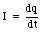(28.1)

where dq is the amount of charge that flows past some given point on the wire during a time period dt. A current of 1 A is equal to 1 C/s. The current density j is defined as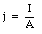(28.2)

where I is the current flowing through the conductor, and A is the cross-sectional area of the conductor. Even though the electrons feel an electric field inside the conductor, they will not accelerate. The electrons will experience significant friction as a result of collisions with the positive ions in the conductor. On average, the electrons will move with a constant speed from the negative terminal of the battery to the positive terminal. Their average velocity, also called the drift velocity vd, is proportional to the electric field E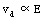(28.3)

For a given density of electrons in the conductor, an increase of the drift velocity of each of the electrons will increase the number of electrons passing by a given point on the conductor per unit of time. This is illustrated in Figure 28.2. During a time interval dt the electrons will cover, on average, a distance equal to dx where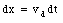(28.4)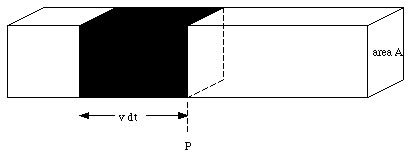Figure 28.2. Motion of average electron in conductor.

All electrons within a distance dx from the point P will therefore pass this point during the time interval dt. Suppose the density of electrons in the conductor is n electrons/m3. The number of electrons dN that will pass P during the time interval dt is then equal to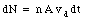(28.5)

Since each electron carries a charge e, the total charge dQ that will pass point P in a time interval dt is equal to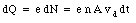(28.6)

The current through the conductor is therefore equal to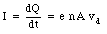(28.7)

Equation (28.7) shows that the current in the conductor is proportional to the cross-sectional area of the conductor and proportional to the drift velocity. Since the drift velocity is proportional to the electric field E the following relation holds for the current in the conductor: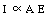(28.8)

The electric field in the conductor is determined by its length L and the potential difference [Delta]V between its two ends (E = [Delta]V/L). Equation (28.8) can therefore be rewritten as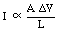(28.9)

Equation (28.9) can be rewritten as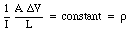(28.10)

The constant of proportionality [rho] is called the resistivity of the material. The resistivity [rho] depends on the characteristics of the conductor ([rho] is small for a good conductor, and [rho] is very large for an insulator). The resistance R of a conductor is defined as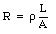(28.11)

The SI unit of resistance is the ohm ([Omega]). Using the resistance R we can rewrite eq.(28.10)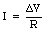(28.12)

Equation (28.12) is called Ohm's Law. Equation (28.12) shows that the current through a conductor is proportional to the potential difference between the ends of the conductor and inversely proportional to its resistance. Equation (28.12) also shows that 1 [Omega] equals 1 V/A.

### Example: Problem 28.5

An aluminum wire has a resistance of 0.10 [Omega]. If you draw this wire through a die, making it thinner and twice as long, what will be its new resistance ?

The initial resistance Ri of the aluminum wire with length L and cross-sectional area A is equal to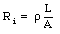(28.13)

The initial volume of the wire is L . A. After passing the wire through the die, it s length has changed to L' and its cross-sectional area is equal A'. Its final volume is therefore equal to L' A'. Since the density of the aluminum does not change, the volume of the wire does not change, and therefore the initial and final dimensions of the wire are related: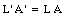(28.14)

or(28.15)

The problem states that the length of the wire is doubled (L' = 2 L). The final cross-sectional area A' is therefore related to the initial cross-sectional area A in the following manner: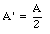(28.16)

The final resistance Rf of the wire is given by(28.17)

The resistance of the wire has increased by a factor of four and is now 0.40 [Omega].

## 28.2. The resistivity of materials

The resistivity [rho] has as units ohm-meter ([Omega] . m). The resistivity of most conductors is between 10-8 [Omega] . m and 10-7 [Omega] . m. The resistivity of a conductor depends not only on the type of the material but also on its temperature. The resistivity of an insulator varies between 1011 [Omega] . m and 1017 [Omega] . m. In all materials the resistivity decreases with decreasing temperature. In some materials, such as lead, zinc, tin and niobium, the resistivity vanishes as the temperature approaches absolute zero. At these low temperatures, these materials exhibit superconductivity.

### Example: Problem 28.17

The air conditioner in a home draws a current of 12 A. Suppose that the pair of wires connecting the air conditioner to the fuse box are No. 10 copper wires with a diameter of 0.259 cm and a length of 25 m each.

a) What is the potential drop along each wire ? Suppose that the voltage delivered to the home is exactly 110 V at the fuse box. What is the voltage delivered to the air conditioner ?

b) Some older homes are wired with No. 12 copper wire with a diameter of 0.205 cm. Repeat the calculation of part (a) for this wire.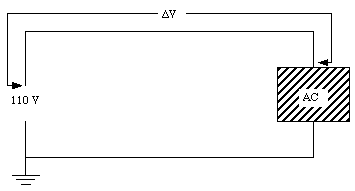Figure 28.3. Wiring diagram of air conditioner in problem 28.17.

a) The resistivity of copper is 1.7 x 10-8 [Omega] . m (see Table 28.1). The resistance RCu of each copper wire is equal to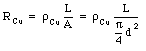(28.18)

where L is the length of the wire and d is its diameter. A current I is flowing through the wires and I = 12 A. The voltage drop [Delta]V across each wire is equal to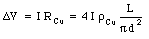(28.19)

Figure 28.3 shows schematically a wiring diagram of the air conditioner circuit. The voltage across the air-conditioner unit is equal to 110 - 2 . [Delta]V, where [Delta]V is given by eq.(28.19). The length of each copper cable is 25 m, and its diameter is equal to 0.259 cm. The voltage drop across each wire is thus equal to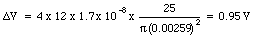(28.20)

The voltage across the AC unit is therefore equal to 108.1 V.

b) A No. 12 wire has a diameter equal to 0.205 cm. The voltage drop across this wire is equal to(28.21)

and the voltage across the AC unit is equal to 106.9 V.

### Example: Problem 28.12

A high voltage transmission line has an aluminum cable of diameter 3.0 cm, 200 km long. What is the resistance of this cable ?

The resistivity of aluminum is 2.8 x 10-8 [Omega] m. the length of the cable is 200 km or 2 x 105 m. The diameter of the cable is 3 cm and its cross-sectional area is equal to [pi] (d/2)2 or 7.1 x 10-4 m2. Substituting these values into eq.(28.11) the resistance of the cable can be determined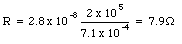(28.22)

## 28.3. Resistance in combination

A device that is specifically designed to have a high resistance is called a resistor. The symbol of a resistor in a circuit diagram is a zigzag line (see Figure 28.4).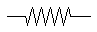Figure 28.4. Symbol of a resistor.

Figure 28.5 shows two resistors with resistance R1 and R2 connected in series. Suppose the current flowing through the circuit is equal to I. The voltage drop [Delta]V1 across resistor R1 is equal to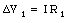(28.23)

and the voltage drop [Delta]V2 across resistor R2 is equal to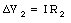(28.24)

The potential difference [Delta]V across the series circuit is equal to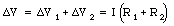(28.25)

Equation (28.25) shows that two resistors connected in series act like one resistor with a resistance equal to the sum of the resistance of resistor 1 and the resistance of resistor 2(28.26)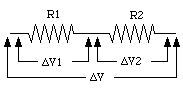Figure 28.5. Two resistors connected in series.

Figure 28.6 shows two resistors connected in parallel. In this circuit, the current through each resistor will be different, but the voltage drop [Delta]V across each resistor will be the same. Using Ohm's law the current I1 flowing through resistor R1 can be calculated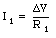(28.27)

and the current I2 flowing through resistor R2 is equal to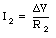(28.28)

The total current flowing through the circuit is equal to the sum of the currents through each resistor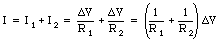(28.29)

The resistor network shown in Figure 28.6 is therefore equivalent to a single resistor R where R can be obtained from the following relation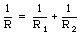(28.30)

Equation (28.30) shows that the resistance of a parallel combination of resistors is always less than the resistance of each of the individual resistors.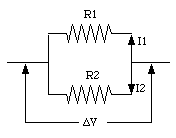Figure 28.6. Two resistor connected in parallel.

### Example: Problem 28.41

Commercially manufactured superconducting cables consist of filaments of superconducting wire embedded in a matrix of copper. As long as the filaments are superconducting, all the current flows in them, and no current flows in the copper. But if the superconductivity suddenly fails because of a temperature increase, the current can spill into the copper; this prevents damage to the filaments of the superconductor. Calculate the resistance per meter of length of a copper matrix. The copper matrix has a diameter of 0.7 mm, and each of the 2100 filaments has a diameter of 0.01 mm.

Consider 1 meter of cable. The cross-sectional area of each filament is [pi] . (d/2)2 = 7.9 x 10-11 m2. The cross-sectional area of 2100 filaments is equal to 1.65 x 10-7 m2. The diameter of the copper matrix is equal to 0.7 mm, and its cross-sectional area is equal to 1.54 x 10-6 m2. The area of the copper itself is thus equal to 1.37 x 10-6 m2. The resistance of the copper matrix per unit length is equal to(28.31)

Suppose the resistivity of the filament at room temperature is the same as the resistivity of copper. The resistance of each superconducting filament is equal to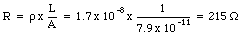(28.32)

The wire can be treated as a parallel circuit of one resistor representing the resistance of the copper matrix and 2100 resistors representing the 2100 strands of superconducting wire. The fraction of the current flowing through the copper matrix can be determined easily. Suppose that the potential difference across the conductor is equal to [Delta]V. The current ICu flowing through the copper matrix is equal to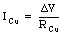(28.33)

The current Ifil flowing through the 2100 filaments is equal to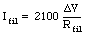(28.34)

The fraction F of the total current flowing through the copper matrix is equal to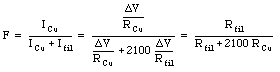(28.35)

Two special cases will need to be considered.

1. The temperature is below the critical temperature. At or below this temperature the resistance of the filaments vanishes (Rfil = 0 [Omega]). Equation (28.35) shows that in this case no current will flow through the copper matrix.

2. If the temperature of the wire is above the critical temperature, the current flow will change drastically. In this case, the fraction of the current flowing through the copper is equal to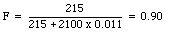(28.36)

The copper matrix will carry 90% of the total current.

### Example: Problem 28.42

What is the resistance of the combination of four resistors shown in Figure 28.7. Each of the resistors has a value of R.Figure 28.7. Problem 28.42.

To find the net resistance of the circuit shown in Figure 28.7, we start calculating the net resistance R34 of the parallel circuit of resistors R3 and R4: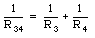(28.37)

or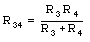(28.38)

The circuit shown in Figure 28.7 is therefore equivalent with the circuit shown in Figure 28.8. Resistors R2 and R34 form a series network and can be replaced by a single resistor with a resistance R234 where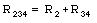(28.39)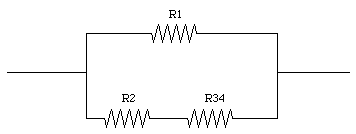Figure 28.8. Problem 28.42.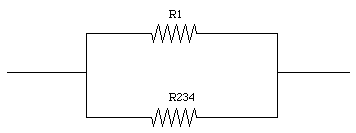Figure 28.9. Problem 28.42.

The circuit shown in Figure 28.8 can now be replaced by an equivalent circuit shown in Figure 28.9. The resistance Rtot of this circuit can be obtained from the following relation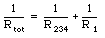(28.40)

or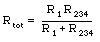(28.41)

In the special case considered, R1 = R2 = R3 = R4 = R. Thus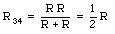(28.42)(28.43)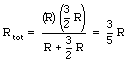(28.44)

For R = 3 [Omega] the total resistance is equal to 1.8 [Omega].

Send comments, questions and/or suggestions via email to wolfs@nsrl.rochester.edu and/or visit the home page of Frank Wolfs.